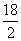## Definition Of Dividend

The number that is divided by another number in a division operation is called a Dividend.

When a company gives its shareholders a part of the profit that it has earned over a period of time, we call that part as dividend. (A part of profit made by a company and given to its shareholders is also called dividend.)

## Example Of DividendHere, a group of 18 triangles is divided into two groups with 9 triangles in each. We write it as =9. Here, 18 is dividend.

A. 758
B. 7
C. 14
D. 104